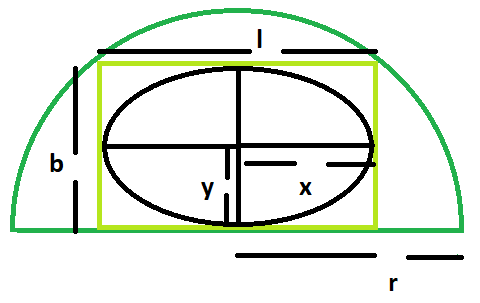GeeksforGeeks App
Open AppBrowser
Continue

# Largest ellipse that can be inscribed within a rectangle which in turn is inscribed within a semicircle

Given here is a semicircle of radius r, which inscribes a rectangle which in turn inscribes an ellipse. The task is to find the area of this largest ellipse.
Examples:

```Input: r = 5
Output: 19.625

Input: r = 11
Output: 94.985```Approach

1. Let the, length of the rectangle = l and breadth of the rectangle = b
2. Let, the length of the major axis of the ellipse = 2x and, the length of the minor axis of the ellipse = 2y
3. As we know, length and breadth of the largest rectangle inside a semicircle are r/√2 and √2r(Please refer here)
4. Also, Area of the ellipse within the rectangle = (π*l*b)/4 = (πr^2/4)

Below is the implementation of above approach

## C++

 `// C++ Program to find the biggest ellipse``// which can be inscribed within a rectangle``// which in turn is inscribed within a semicircle` `#include ``using` `namespace` `std;` `// Function to find the area``// of the biggest ellipse``float` `ellipsearea(``float` `r)``{` `    ``// the radius cannot be negative``    ``if` `(r < 0)``        ``return` `-1;` `    ``// area of the ellipse``    ``float` `a = (3.14 * r * r) / 4;` `    ``return` `a;``}` `// Driver code``int` `main()``{``    ``float` `r = 5;``    ``cout << ellipsearea(r) << endl;``    ``return` `0;``}`

## Java

 `// Java Program to find the biggest ellipse``// which can be inscribed within a rectangle``// which in turn is inscribed within a semicircle``class` `GFG``{` `// Function to find the area``// of the biggest ellipse``static` `float` `ellipsearea(``float` `r)``{` `    ``// the radius cannot be negative``    ``if` `(r < ``0``)``        ``return` `-``1``;` `    ``// area of the ellipse``    ``float` `a = (``float``)((``3``.14f * r * r) / ``4``);` `    ``return` `a;``}` `// Driver code``public` `static` `void` `main(String[] args)``{``    ``float` `r = ``5``;``    ``System.out.println(ellipsearea(r));``}``}` `// This code is contributed by Code_Mech.`

## Python3

 `# Python3 Program to find the biggest ellipse``# which can be inscribed within a rectangle``# which in turn is inscribed within a semicircle` `# Function to find the area of``# the biggest ellipse``def` `ellipsearea(r) :` `    ``# the radius cannot be negative``    ``if` `(r < ``0``) :``        ``return` `-``1``;` `    ``# area of the ellipse``    ``a ``=` `(``3.14` `*` `r ``*` `r) ``/` `4``;` `    ``return` `a;` `# Driver code``if` `__name__ ``=``=` `"__main__"` `:` `    ``r ``=` `5``;``    ``print``(ellipsearea(r));` `# This code is contributed by Ryuga`

## C#

 `// C# Program to find the biggest ellipse``// which can be inscribed within a rectangle``// which in turn is inscribed within a semicircle``using` `System;``class` `GFG``{` `// Function to find the area``// of the biggest ellipse``static` `float` `ellipsearea(``float` `r)``{` `    ``// the radius cannot be negative``    ``if` `(r < 0)``        ``return` `-1;` `    ``// area of the ellipse``    ``float` `a = (``float``)((3.14 * r * r) / 4);` `    ``return` `a;``}` `// Driver code``public` `static` `void` `Main()``{``    ``float` `r = 5;``    ``Console.WriteLine(ellipsearea(r));``}``}` `// This code is contributed by Akanksha Rai`

## PHP

 ``

## Javascript

 ``

Output:

`19.625`

Time Complexity: O(1)
Auxiliary Space: O(1)

My Personal Notes arrow_drop_up